## Want to keep learning?

This content is taken from the Raspberry Pi Foundation & National Centre for Computing Education's online course, How Computers Work: Demystifying Computation. Join the course to learn more.
2.2

## Raspberry Pi Foundation

Skip to 0 minutes and 0 seconds In this step, we’re going to have a look at how transistors can be combined together to form what are called logic gates. And in particular, we’ll look at the NAND gate. We’ve already seen how transistors can be used to store the value 1 or 0 by acting like a simple switch. We’re now going to look at combining those transistors together so they can perform more complex operations. Here, we have a circuit set up containing two transistors. Again, you don’t have to understand what is going on in this circuit, just as long as you can understand the basic inputs and outputs. The two switches are acting as inputs to the transistor setup, and the LED represents the output.

Skip to 0 minutes and 39 seconds At the moment, when both inputs are in the off position, the LED is on. Either of the inputs can be turned on, leaving the other one off, and the LED will stay on. It’s only when both inputs are turned on that the LED will switch off. This arrangement of transistors is called a NAND gate, and you’ll learn about why later. Now, rather than drawing two transistors every time we want to represent a NAND gate, we can use a symbol that looks like this. Whether we’re using the symbol or the two transistors, the inputs and outputs remain the same. When both inputs are off, the output is on. Turning either of the inputs on doesn’t affect the output.

Skip to 1 minute and 22 seconds And only when both inputs are in the on position does the output become off.

Skip to 1 minute and 29 seconds We can use this to draw what is called a truth table. With both inputs at 0 and 0, the lamp is on, so the output is 1. If either of the becomes 1, the lamp remains on, so the output remains 1. Only when both inputs are 1 does the output change to 0. So this is the truth table for a NAND gate. You can play with your own simulation of a NAND gate on the website circuitverse.org. Here you can select different gates. You can select inputs, which can be a 1 or a 0, and outputs in the form of a digital LED.

Skip to 2 minutes and 11 seconds This will allow you to play around with a NAND gate and have a look at the truth table for yourself. You could even have a go to experimenting with different types of gates, which we’ll be covering in the next step.

# Creating NAND gates

In this step, we’re going to have a look at how transistors can be combined together to form what are called logic gates, and in particular we’ll look at the NAND gate.

We’ve already seen how transistors can be used to represent the value 1 or 0 by acting like simple switches. We’re now going to look at combining these transistors together so they can perform more complex operations.

The diagram below shows a circuit containing two transistors. Again you don’t have to understand what is going on in this circuit, just as long as you can understand the basic inputs and outputs.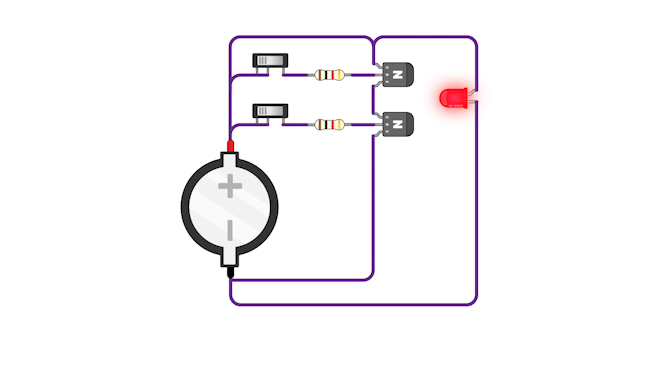The two switches are acting as inputs to the transistor setup, and the LED represents the output. When both switches are in the off position, the LED is on. Either of the switches can be turned on, leaving the other one off, and the LED still stays on. It’s only when both switches are turned on that the LED will switch off.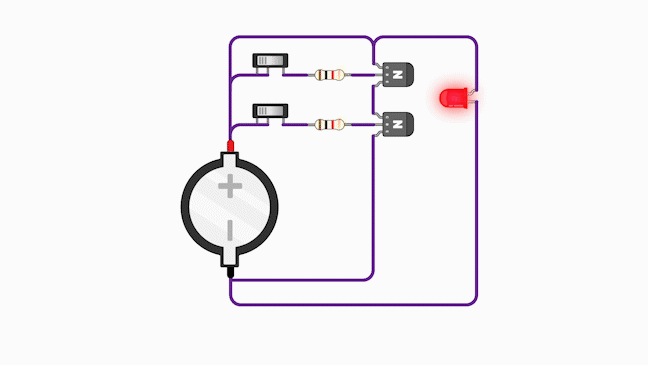This arrangement of transistors is called a NAND gate, and you’ll learn about why later.

Now, rather than drawing two transistors every time we want to represent a NAND gate, we can use a symbol that looks like this.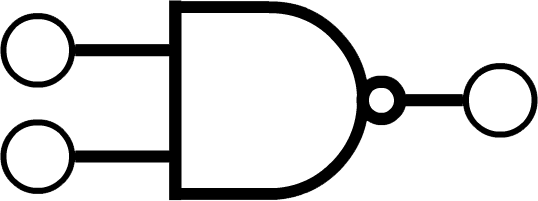Whether we use this symbol or show the two transistors, the behaviour of the NAND gate is the same. When both switches are off, the output is on. Turning either of the switches individually doesn’t effect the output. Only when both switches are in the “on” position does the output become “off”.
We can represent this behaviour by drawing what is called a truth table, where the state of the switches is represented by 1s and 0s. When both switches are off, the inputs are both 0. Since the lamp is on, the output in this state is 1. If we turn one of the switches to the “on” position, the corresponding input is 1; the lamp remains on, so the output is 1. Only when both inputs are one does the output change to 0.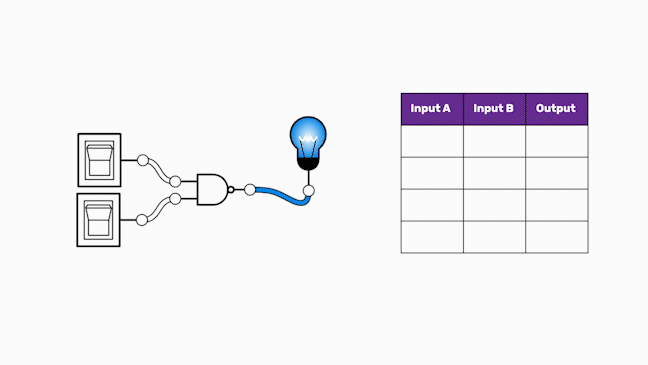So the full truth table for a NAND gate is as shown below.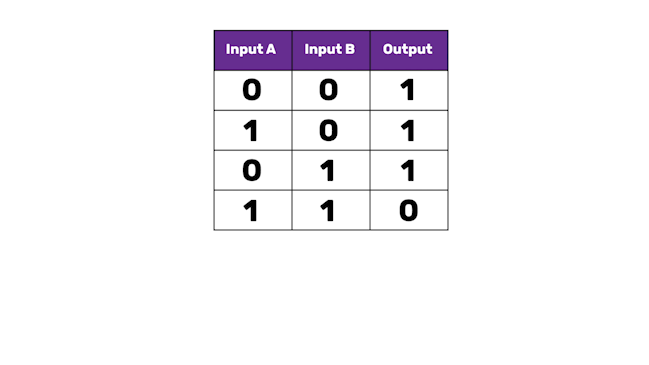You can play with your own simulation of a NAND gate on the website CircuitVerse.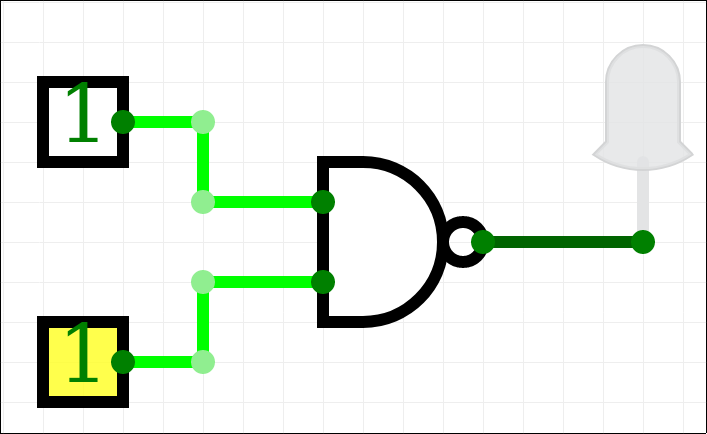Here you can select different gates, and choose inputs which can be 1 or 0, with outputs in the form of a digital LED. This will allow you to play around with a NAND gate and investigate the truth table for yourself.

You could even have a go at experimenting with different types of gate, which we will be covering in the next step.